# 【三維路徑規劃】基於matlab RRT演算法無人機路徑規劃【含Matlab原始碼 155期】

## 一、A_star演算法簡介

0 引言 隨著現代技術的發展，飛行器種類不斷變多，應用也日趨專一化、完善化，如專門用作植保的大疆PS-X625無人機，用作街景拍攝與監控巡察的寶雞行翼航空科技的X8無人機，以及用作水下救援的白鯊MIX水下無人機等，決定飛行器效能主要是內部的飛控系統和外部的路徑規劃問題。就路徑問題而言，在具體實施任務時僅靠操作員手中的遙控器控制無人飛行器執行相應的工作，可能會對操作員心理以及技術提出極高的要求，為了避免個人操作失誤，進而造成飛行器損壞的危險，一種解決問題的方法就是對飛行器進行航跡規劃。 飛行器的測量精度，航跡路徑的合理規劃，飛行器工作時的穩定性、安全性等這些變化對飛行器的綜合控制系統要求越來越高。無人機航路規劃是為了保證無人機完成特定的飛行任務，並且能夠在完成任務的過程中躲避各種障礙、威脅區域而設計出最優航跡路線的問題。常見的航跡規劃演算法如圖1所示。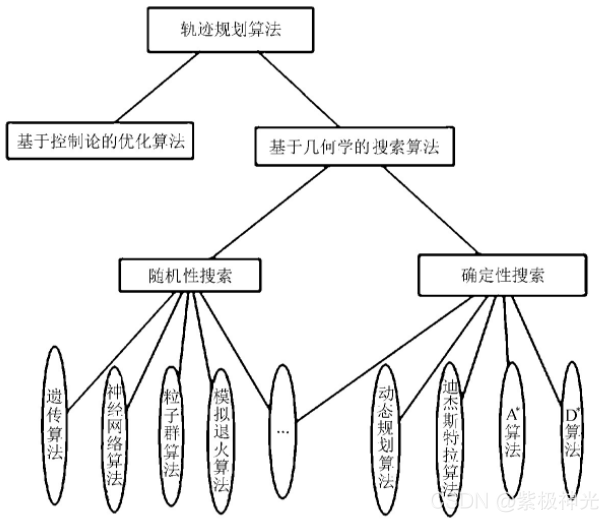圖1 常見路徑規劃演算法 文中主要對無人機巡航階段的航跡規劃進行研究，假設無人機在飛行中維持高度與速度不變，那麼航跡規劃成為一個二維平面的規劃問題。在航跡規劃演算法中,A演算法計算簡單，容易實現。在改進A演算法基礎上，提出一種新的、易於理解的改進A演算法的無人機航跡規劃方法。傳統A演算法將規劃區域柵格化，節點擴充套件只限於柵格線的交叉點，在柵格線的交叉點與交叉點之間往往存在一定角度的兩個運動方向。將存在角度的兩段路徑無限放大、細化，然後分別用兩段上的相應路徑規劃點作為切點，找到相對應的組成內切圓的圓心，然後作弧，並求出相對應的兩切點之間的弧所對應的圓心角，根據下式計算出弧線的長度式中：R———內切圓的半徑； α———切點之間弧線對應的圓心角。

## 二、RRT演算法簡介

1 RRT定義 RRT（Rapidly-Exploring Random Tree）演算法是一種基於取樣的路徑規劃演算法，常用於移動機器人路徑規劃，適合解決高維空間和複雜約束下的路徑規劃問題。基本思想是以產生隨機點的方式通過一個步長向目標點搜尋前進，有效躲避障礙物，避免路徑陷入區域性極小值，收斂速度快。本文通過matlab實現RRT演算法，解決二維平面的路徑規劃問題。 2 地圖 為了方便演算法的實現，使用離散量來表達環境地圖。其中，數值0表示無障礙物的空區域，數值1表示該區域有障礙物。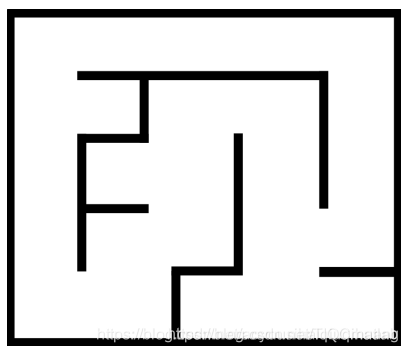RRT演算法中搜索到的頂點座標為連續點，在地圖中產生隨機點，演算法將通過連續的點構建樹。此過程中，對樹枝和頂點進行檢測，檢測頂點所處位置是否是空區域。下載附錄中.dat檔案，繪製地圖。 ```c colormap=[1 1 1; 0 0 0; 1 0 0; 0 1 0; 0 0 1]; imshow(uint8(map),colormap)

``` note:資料中的列為x軸，行為y軸

3 RRT演算法原理 通過matlab程式構建從起始位置到目標位置的樹，並生成連線兩個點的路徑。使用一顆中心點在起始點的樹，而不是兩顆樹（一箇中心點在起始位置，一箇中心點在目標位置）。 編寫一個matlab函式，輸入和輸出有相同的形式。

```c function [vertices, edges, path] = rrt(map, q_start, q_goal, k, delta_q, p)

```

4 障礙物檢測 檢測樹枝（即q_near和q_new之間的edge）是否處於自由空間，可以使用增量法或者等分法，示意圖如下(假設兩點之間有10個點，左圖為為增量檢測法，右圖為等分法，從示意圖中可以看出使用等分法檢測次數更少)：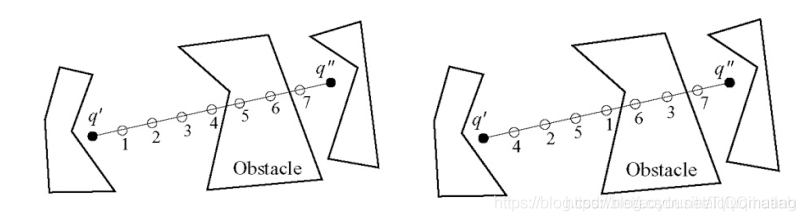在本文中，使用k=10000，delta_q=50,p=0.3, 我們將獲得如下結果：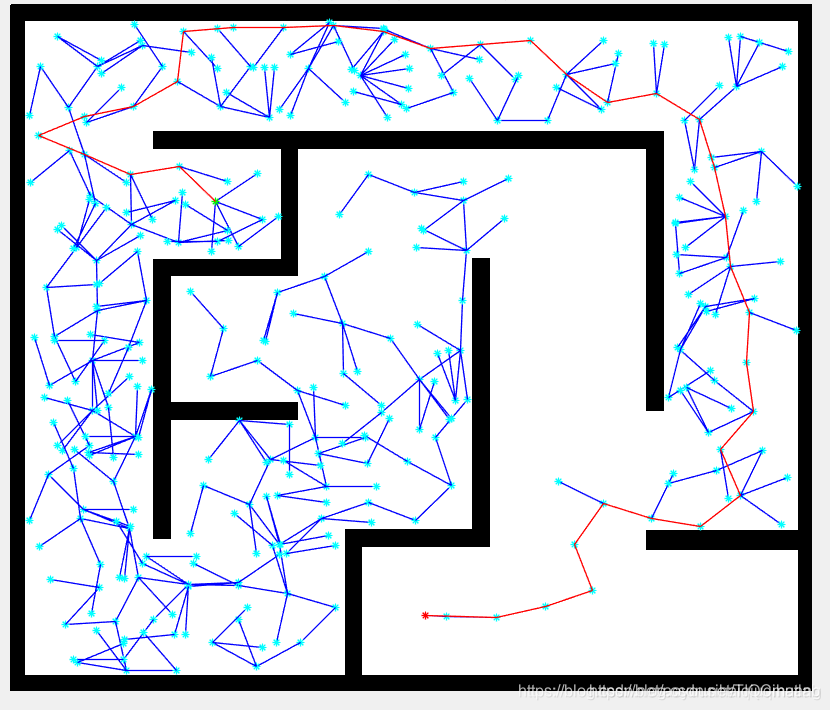5 路徑平滑處理 完成基本的RRT演算法之後，我們獲得了一條從起點到終點的路徑，現在對這條路徑進行平滑和降噪處理，處理完成之後，我們將得到一條更短的路徑。 採用貪心演算法： 連線q_start和q_goal,如果檢測到兩個點之間有障礙物，則將q_goal替換為前一個點，直到兩個點能連線上（中間無障礙物）為止。一旦q_goal被連線上， 在matlab中定義平滑函式：

```c function [path_smooth] = smooth(map, path, vertices, delta)

``` 其中： path: 從起始點到目標位置的路徑索引號 vertices：樹中所有的頂點座標 delta：增量距離，用來檢測路徑頂點之間的直接連線是否在自由空間之內，每個edge都被delta分割成幾段 path_smooth：經過平滑處理之後，路徑點將會減少，用path_smooth記錄平滑之後的路徑,仍然是一個行向量，記錄路徑的索引號

## 三、部分原始碼

```c clearvars close all x_max = 640; y_max = 480; z_max = 400;

EPS = 20; numNodes = 2000;

q_start.coord = [0 0 0]; q_start.cost = 0; q_start.parent = 0; q_goal.coord = [640 400 180]; q_goal.cost = 0;

nodes(1) = q_start; figure(1)

for i = 1:1:numNodes q_rand = [rand(1)x_max rand(1)y_max rand(1)*z_max]; plot3(q_rand(1), q_rand(2), q_rand(3), 'x', 'Color', [0 0.4470 0.7410])

``````% Break if goal node is already reached
for j = 1:1:length(nodes)
if nodes(j).coord == q_goal.coord
break
end
end

% Pick the closest node from existing list to branch out from
ndist = [];
for j = 1:1:length(nodes)
n = nodes(j);
tmp = dist_3d(n.coord, q_rand);
ndist = [ndist tmp];
end
[val, idx] = min(ndist);
q_near = nodes(idx);

q_new.coord = steer3d(q_rand, q_near.coord, val, EPS);
line([q_near.coord(1), q_new.coord(1)], [q_near.coord(2), q_new.coord(2)], [q_near.coord(3), q_new.coord(3)], 'Color', 'k', 'LineWidth', 2);
drawnow
hold on
q_new.cost = dist_3d(q_new.coord, q_near.coord) + q_near.cost;

% Within a radius of r, find all existing nodes
q_nearest = [];
r = 50;
neighbor_count = 1;
for j = 1:1:length(nodes)
if (dist_3d(nodes(j).coord, q_new.coord)) <= r
q_nearest(neighbor_count).coord = nodes(j).coord;
q_nearest(neighbor_count).cost = nodes(j).cost;
neighbor_count = neighbor_count+1;
end
end

% Initialize cost to currently known value
q_min = q_near;
C_min = q_new.cost;

% Iterate through all nearest neighbors to find alternate lower
% cost paths

for k = 1:1:length(q_nearest)
if q_nearest(k).cost + dist_3d(q_nearest(k).coord, q_new.coord) < C_min
q_min = q_nearest(k);
C_min = q_nearest(k).cost + dist_3d(q_nearest(k).coord, q_new.coord);
line([q_min.coord(1), q_new.coord(1)], [q_min.coord(2), q_new.coord(2)], [q_min.coord(3), q_new.coord(3)], 'Color', 'g');
hold on
end
end

% Update parent to least cost-from node
for j = 1:1:length(nodes)
if nodes(j).coord == q_min.coord
q_new.parent = j;
end
end

% Append to nodes
nodes = [nodes q_new];
``````

end

D = []; for j = 1:1:length(nodes) tmpdist = dist_3d(nodes(j).coord, q_goal.coord); D = [D tmpdist]; end

% Search backwards from goal to start to find the optimal least cost path [val, idx] = min(D); q_final = nodes(idx); q_goal.parent = idx; q_end = q_goal; nodes = [nodes q_goal]; while q_end.parent ~= 0

``````hold on
q_end = nodes(start);
``````

end function d = dist_3d(q1,q2) d = sqrt((q1(1)-q2(1))^2 + (q1(2)-q2(2))^2 + (q1(3)-q2(3))^2); end

function A = steer(qr, qn, val, eps) qnew = [0 0]; if val >= eps qnew(1) = qn(1) + ((qr(1)-qn(1))eps)/dist_3d(qr,qn); qnew(2) = qn(2) + ((qr(2)-qn(2))eps)/dist_3d(qr,qn); qnew(3) = qn(3) + ((qr(3)-qn(3))*eps)/dist_3d(qr,qn); else qnew(1) = qr(1); qnew(2) = qr(2); qnew(3) = qr(3); end

```

## 四、執行結果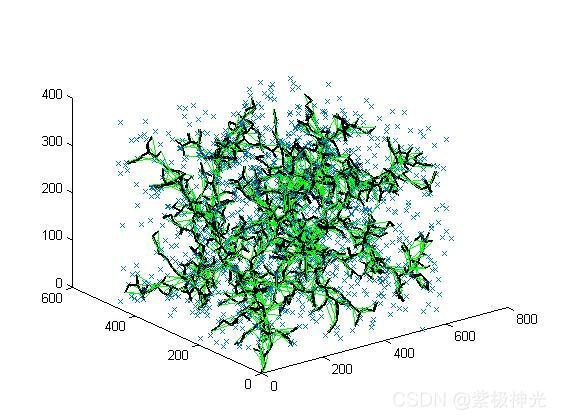## 五、matlab版本及參考文獻

1 matlab版本 2014a

2 參考文獻  包子陽,餘繼周,楊杉.智慧優化演算法及其MATLAB例項（第2版）[M].電子工業出版社，2016. 張巖,吳水根.MATLAB優化演算法原始碼[M].清華大學出版社，2017. RRT路徑規劃演算法